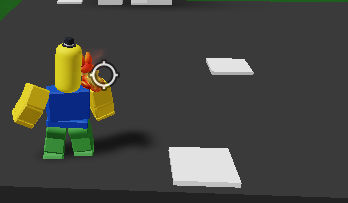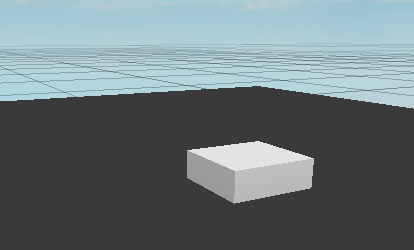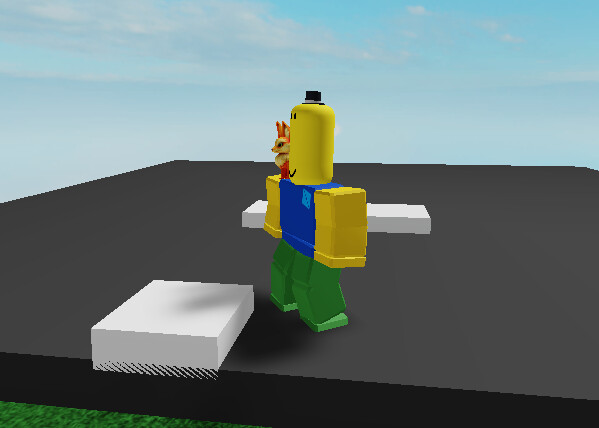# Part clipping through plot

``````local function snapToGrid(position : Vector3)

return Vector3.new(math.floor(position.X / grid_Size + 0.5) * grid_Size, math.floor(position.Y / grid_Size + 0.5), math.floor(position.Z / grid_Size + 0.5))

end

local function snapToGrid(position : Vector3)
return Vector3.new(math.floor(position.X / grid_Size + 0.5) * grid_Size, math.floor(position.Y / grid_Size + 0.5), math.floor(position.Z / grid_Size + 0.5))
end

function Placement.PositionPartOnGrid(part : BasePart, mouse : Mouse)
part.Position = snapToGrid(mouse.Hit.Position)
end
``````

I’ve made a placement system and need some methods to fix part clipping through the plot.I’m guessing the white Parts are your placed items and the medium grey Part is the plot?
What exactly do you mean by “clipping through the plot” since 1 picture doesn’t really explain your issue very well.

If that’s all one script why do you have the same `snapToGrid function` twice?

Yes, the medium grey part is the plot. “why do you have the same `snapToGrid function` twice?” That’s just a a typo.

What I mean is that the white part is being placed a little under the plot.

What I want is the white part to be placed like this:Isn’t it because when you put `math.floor(position.Y / grid_Size + 0.5)` you are just putting it at .5 plus the position.Y divided by the grid_Size?
Shouldn’t it be `math.floor(position.Y / grid_Size + grid_Size/2)` to get half the height of the grid part you are placing added to the Y Position?

That doesn’t fix the problem, it’s the grid formula.

The PositionPartOnGrid formula is telling the Part to go to the Position returned by the snapToGrid function’s formula. You need to fix one of them to place the Part half it’s height higher on the Y axis.

Yes, I tried placing it half of it’s height but still no luck.

``````part.Position = snapToGrid(mouse.Hit.Position) + Vector3.new(0, part.Size.Y / 2, 0)
``````

And how does it appear with that script?1 Like

You could put a print() line in after each to see what the values are for the read Position and the placed Position when you do the calculations.
It almost looks like the Part is getting placed 1/4 of it’s height too low. Maybe check it with different sized Parts to see if it’s the read Position calculation or the placed Position calculation that are getting messed up.

I’ve found a solution. What I did was use this formula: ` part1.Size.Y / 2 + part2.Size.Y / 2 + part1.Position.Y`

1 Like